1. /
2. CBSE
3. /
4. Class 07
5. /
6. Mathematics
7. /
8. NCERT Solutions for Class...

# NCERT Solutions for Class 7 Maths Exercise 8.2### myCBSEguide App

Download the app to get CBSE Sample Papers 2023-24, NCERT Solutions (Revised), Most Important Questions, Previous Year Question Bank, Mock Tests, and Detailed Notes.

NCERT solutions for Maths Comparing Quantities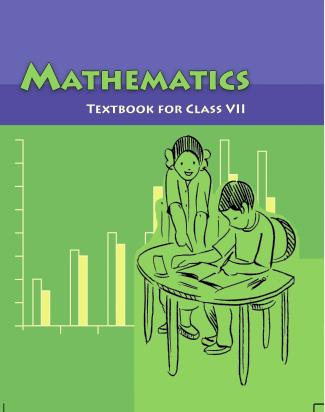## NCERT Solutions for Class 7 Maths Comparing Quantities

###### Question 1.Convert the given fractional numbers to percent:

(a) {tex}\frac{1}{8}{/tex}

(b) {tex}\frac{5}{4}{/tex}

(c) {tex}\frac{3}{{40}}{/tex}

(d) {tex}\frac{2}{7}{/tex}

(a) {tex}\frac{1}{8}{/tex} = {tex}\frac{1}{8} \times 100\% = \frac{{25}}{2}\% {/tex} = 12.5%

(b) {tex}\frac{5}{4}{/tex} = {tex}\frac{5}{4} \times 100\% = {/tex} 5 x 25% = 125%

(c) {tex}\frac{3}{{40}}{/tex} = {tex}\frac{3}{{40}} \times 100\% = \frac{3}{2} \times 5\% = \frac{{15}}{2}\% {/tex} = 7.5%

(d) {tex}\frac{2}{7}{/tex} = {tex}\frac{2}{7} \times 100\% = \frac{{200}}{7}\% = 28\frac{4}{7}\% {/tex}

NCERT Solutions for Class 7 Maths Exercise 8.2

###### Question 2.Convert the given decimal fractions to percents:

(a) 0.65

(b) 2.1

(c) 0.02

(d) 12.35

(a) 0.65 = {tex}\frac{{65}}{{100}} \times 100\% {/tex} = 65%

(b) 0.02 = {tex}\frac{2}{{100}} \times 100\% {/tex} = 2%

NCERT Solutions for Class 7 Maths Exercise 8.2

###### Question 3.Estimate what part of the figures is coloured and hence find the percent which is coloured: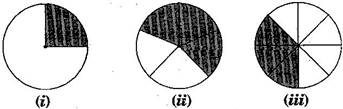(i) Coloured part = {tex}\frac{1}{4}{/tex}

{tex}\therefore {/tex} Percent of coloured part = {tex}\frac{1}{4} \times 100\% {/tex} = 25%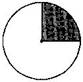(ii) Coloured part = {tex}\frac{3}{5}{/tex}

{tex}\therefore {/tex} Percent of coloured part = {tex}\frac{3}{5} \times 100\% {/tex} = 60%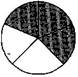(iii) Coloured part = {tex}\frac{3}{8}{/tex}

{tex}\therefore {/tex} Percent of coloured part = {tex}\frac{3}{8} \times 100\% {/tex} = {tex}\frac{3}{2} \times 25\% {/tex}

= 37.5%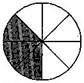###### Question 4.Find:

(a) 15% of 250

(b) 1% of 1 hour

(c) 20% of Rs. 2500

(d) 75% of 1 kg

(a) 15% of 250 = {tex}\frac{{15}}{{100}} \times 250{/tex} = 15 x 2.5 = 37.5

(b) 1% of 1 hours = 1% of 60 minutes = 1% of (60 x 60) seconds

= {tex}\frac{1}{{100}} \times 60 \times 60{/tex} = 6 x 6 = 36 seconds

(c) 20% of Rs. 2500 = {tex}\frac{{20}}{{100}} \times 2500{/tex} = 20 x 25 = Rs. 500

(d) 75% of 1 kg = 75% of 1000 g = {tex}\frac{{75}}{{100}} \times 1000{/tex} = 750 g = 0.750 kg

NCERT Solutions for Class 7 Maths Exercise 8.2

###### Question 5.Find the whole quantity if:

(a) 5% of it is 600

(b) 12% of it is Rs. 1080

(c) 40% of it is 500 km

(d) 70% of it is 14 minutes

(e) 8% of it is 40 liters

Let the whole quantity be {tex}x{/tex} in given questions:

(a) 5% of {tex}x{/tex} = 600 {tex} \Rightarrow {/tex} {tex}\frac{5}{{100}} \times x = 600{/tex}

{tex} \Rightarrow {/tex} {tex}x = \frac{{600 \times 100}}{5}{/tex} = 12,000

(b) 12% of {tex}x{/tex} = Rs. 1080 {tex} \Rightarrow {/tex}{tex}\frac{{12}}{{100}} \times x = 1080{/tex}

{tex} \Rightarrow {/tex} {tex}x = \frac{{1080 \times 100}}{{12}}{/tex} = Rs. 9,000

(c) 40% of {tex}x{/tex} = 500 km {tex} \Rightarrow {/tex} {tex}\frac{{40}}{{100}} \times x = 500{/tex}

{tex} \Rightarrow {/tex} {tex}x = \frac{{500 \times 100}}{{40}}{/tex} = 1,250 km

(d) 70% of {tex}x{/tex} = 14 minutes {tex} \Rightarrow {/tex} {tex}\frac{{70}}{{100}} \times x = 14{/tex}

{tex} \Rightarrow {/tex} {tex}x = \frac{{14 \times 100}}{{70}}{/tex} = 20 minutes

(e) 8% of {tex}x{/tex} = 40 liters {tex} \Rightarrow {/tex} {tex}\frac{8}{{100}} \times x = 40{/tex}

{tex} \Rightarrow {/tex} {tex}x = \frac{{40 \times 100}}{8}{/tex} = 500 liters

NCERT Solutions for Class 7 Maths Exercise 8.2

###### Question 6.Convert given percents to decimal fractions and also to fractions in simplest forms:

(a) 25%

(b) 150%

(c) 20%

(d) 5%

 S. No. Percents Fractions Simplest form Decimal form (a) 25% {tex}\frac{{25}}{{100}}{/tex} {tex}\frac{1}{4}{/tex} 0.25 (b) 150% {tex}\frac{{150}}{{100}}{/tex} {tex}\frac{3}{2}{/tex} 1.5 (c) 20% {tex}\frac{{20}}{{100}}{/tex} {tex}\frac{1}{5}{/tex} 0.2 (d) 5% {tex}\frac{5}{{100}}{/tex} {tex}\frac{1}{{20}}{/tex} 0.05

NCERT Solutions for Class 7 Maths Exercise 8.2

###### Question 7.In a city, 30% are females, 40% are males and remaining are children. What percent are children?

Given: Percentage of females = 30%

Percentage of males = 40%

Total percentage of females and males = 30 + 40 = 70%

Percentage of children = Total percentage – Percentage of males and females

= 100% – 70%

= 30%

Hence, 30% are children.

NCERT Solutions for Class 7 Maths Exercise 8.2

###### Question 8.Out of 15,000 voters in a constituency, 60^ voted. Find the percentage of voters who did not vote. Can you now find how many actually did not vote?

Total voters = 15,000

Percentage of voted candidates = 60%

Percentage of not voted candidates = 100 – 60 = 40%

Actual candidates, who did not vote = 40% of 15000

= {tex}\frac{{40}}{{100}} \times 15000{/tex} = 6,000

Hence, 6,000 candidates did not vote.

NCERT Solutions for Class 7 Maths Exercise 8.2

###### Question 9.Meeta saves Rs. 400 from her salary. If this is 10% of her salary. What is her salary?

Let Meera’s salary be Rs.{tex}x.{/tex}

Now, 10% of salary = Rs. 400

{tex} \Rightarrow {/tex} 10% of {tex}x{/tex} = Rs. 400

{tex} \Rightarrow {/tex} {tex}\frac{{10}}{{100}} \times x = 400{/tex}

{tex} \Rightarrow {/tex} {tex}x = \frac{{400 \times 100}}{{10}}{/tex}

{tex} \Rightarrow {/tex} {tex}x = {/tex} 4,000

Hence, Meera’s salary is Rs. 4,000.

NCERT Solutions for Class 7 Maths Exercise 8.2

###### Question 10.A local cricket team played 20 matches in one season. It won 25% of them. How many matches did they win?

Number of matches played by cricket team = 20

Percentage of won matches = 25%

Total matches won by them = 25% of 20

= {tex}\frac{{25}}{{100}} \times 20{/tex}

= 5

Hence, they won 5 matches.

## NCERT Solutions for Class 7 Maths Exercise 8.2

NCERT Solutions Class 7 Maths PDF (Download) Free from myCBSEguide app and myCBSEguide website. Ncert solution class 7 Maths includes text book solutions from Class 7 Maths Book . NCERT Solutions for CBSE Class 7 Maths have total 15 chapters. 7 Maths NCERT Solutions in PDF for free Download on our website. Ncert Maths class 7 solutions PDF and Maths ncert class 7 PDF solutions with latest modifications and as per the latest CBSE syllabus are only available in myCBSEguide.

## CBSE app for Students

To download NCERT Solutions for Class 7 Maths, Social Science Computer Science, Home Science, Hindi English, Maths Science do check myCBSEguide app or website. myCBSEguide provides sample papers with solution, test papers for chapter-wise practice, NCERT solutions, NCERT Exemplar solutions, quick revision notes for ready reference, CBSE guess papers and CBSE important question papers. Sample Paper all are made available through the best app for CBSE students and myCBSEguide website.Test Generator

Create question paper PDF and online tests with your own name & logo in minutes.myCBSEguide

Question Bank, Mock Tests, Exam Papers, NCERT Solutions, Sample Papers, Notes

### 3 thoughts on “NCERT Solutions for Class 7 Maths Exercise 8.2”

1. It is very good and useful for students. When you not goted your answer then you help this sheet.

2. Thanks for solve help

3. Really its very good for students